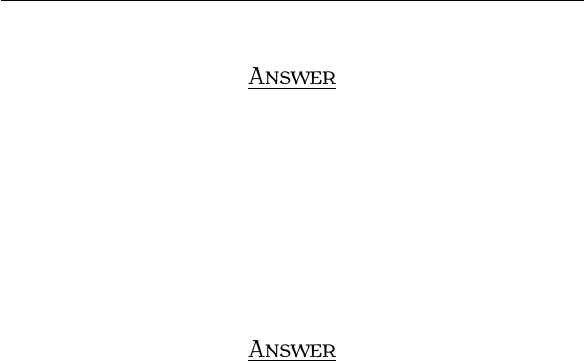Study Guides (400,000)
US (230,000)
BC (3,000)
MATH (90)
All (80)
Midterm

# Exam3210Sample3AnsExam

Department
Mathematics
Course Code
MATH 2211
Professor
All
Study Guide
Midterm

This preview shows pages 1-2. to view the full 6 pages of the document.MT T  S 
I S. Y
A , 
Q . THE PROPERTIES OF DETERMINANTS
a. An n×nmatrix Ais called orthogonal if AAt=I. If Ais orthogonal show that det(A) = ±1.
A
By the properties of determinant, det(AAt) = det(A)det(At) = det(A)det(A) = det(A)2=det(I) = 1. So,
det(A) = ±1.
b. An n×nmatrix A is called skew-symmetric if At=A. Show that if Ais skew-symmetric and nis
an odd positive integer, then Ais not invertible.
A
By the properties of determinant, det(At) = det(A)Ñdet(A) = det(A)Ñdet(A) = (1)ndet(A)Ñ
det(A) = det(A). So, we get det(A) = 0 which implies that Ais not invertible. Note that Ameans that
EVERY ROW of Ais multiplied by -1.
1

Only pages 1-2 are available for preview. Some parts have been intentionally blurred.Q . CRAMER’S RULE, VOLUME, AND LINEAR TRANSFORMATIONS
Find the volume of the parallelepiped with one vertex at the origin and adjacent vertices are (1,0,2),
(1,2,4), and (7,1,0).
A
We need calculate the determinant
117
021
240
,
then take the absolute value to get the volume. So, the volume of the parallelepiped is |22|= 22.
2